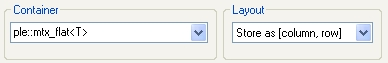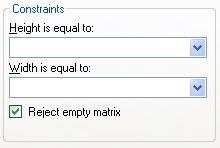XLL+ Class Library (7.0)

# Flat layout

### Matrix argument laid out as a continous array of values

To demonstrate the use of a different matrix layout, we are going to add a function which returns the location of the maximum value within a two-dimensional array. Add the simple function shown below to the end of Tutorial1.cpp:CopyC++
```#include <algorithm>

// Find location of maximum value in adValues
// adValues must be a continuous array, with columns held together
int FindMax(int cCols, int cRows, const double *adValues, int* pnMaxCol,
int* pnMaxRow)
{
const double* pdEnd = adValues + (cRows * cCols);
// Use STL algorithm to find offset of max value
const double* pdMax = std::max_element(adValues, pdEnd);
if (pdMax == pdEnd)
return 0;
// Translate offset to coords
*pnMaxRow = (int)((pdMax - adValues) % cRows);
*pnMaxCol = (int)((pdMax - adValues) / cRows);
return 1;
}```

As you can see, this function takes advantage of the STL max_element algorithm to do all the hard work. The important things to note, as far as our add-in function is concerned, are:

• the algorithm expects data to be in a continous array of values;
• we have (arbitrarily) decided that they should be stored with columns held together.

Next, we'll use the XLL+ Function Wizard to apply these layout rules.

### Create the function

Using the Function Wizard, add a new function, with properties as shown below:

 Name: MAXCOORD Return type: CXlOper Category: Statistical Description: Get the x or y coordinate of the maximum value in an array

### Add a Matrix argument

Add the array argument, as follows:

 Type: Double[][] Name: Array Description: 2-dimensional array of numbers

### Set the Matrix layout

Open the Argument Editor for Array, and switch to the Matrix tab.

Set the following fields:

 Container: ple::mtx_flat Layout: Store as [column, row]### Defend against empty arrays

The code in the add-in function may blow up if the array is empty, so defend against this by setting the "Reject empty matrix" flag:

 Reject empty matrix: trueIf the user enters an empty array, then an exception will be thrown by the validation code, and an error message such as `"#ERROR: Expected at least one cell for Array"` will be returned. If there is any code that assumes Array to be non-empty, e.g:CopyC++
`CallFunction(&Array);`

orCopyC++
`CallFunction(Array.begin());`

it will not be called, because the function has already ended.

Click OK to close the Argument Editor.

### Add a Boolean argument

In the Function Wizard add a new argument, XCoord, with fields as follows:

 Name: XCoord Type: Boolean Dimensions: Scalar Description: True for the x coordinate (column number), false for y (row number)Note that XCoord is a Boolean argument (i.e. True or False). It will be passed to our add-in function as type BOOL (int), with values TRUE (1) or FALSE (0).

Click on OK to return to Visual Studio and complete the code.